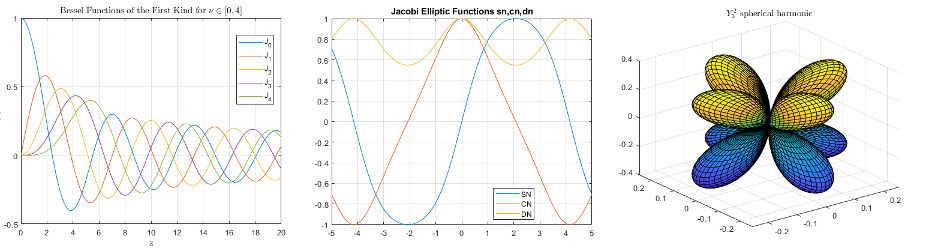# Special Functions

Bessel, Legendre, elliptic, error, gamma, and other functions

Special functions are a group of well-known mathematical functions that frequently arise in real-world applications. You can use them to calculate Bessel functions, beta functions, gamma functions, error functions, elliptic integrals, and more. Since the properties of these functions have been studied extensively, you can find more information about many of them in the NIST Digital Library of Mathematical Functions.## Functions

expand all

 `airy` Airy Functions `besselh` Bessel function of third kind (Hankel function) `besseli` Modified Bessel function of first kind `besselj` Bessel function of first kind `besselk` Modified Bessel function of second kind `bessely` Bessel function of second kind
 `beta` Beta function `betainc` Incomplete beta function `betaincinv` Beta inverse cumulative distribution function `betaln` Logarithm of beta function
 `erf` Error function `erfc` Complementary error function `erfcinv` Inverse complementary error function `erfcx` Scaled complementary error function `erfinv` Inverse error function
 `gamma` Gamma function `gammainc` Incomplete gamma function `gammaincinv` Inverse incomplete gamma function `gammaln` Logarithm of gamma function `psi` Digamma and polygamma functions
 `ellipj` Jacobi elliptic functions `ellipke` Complete elliptic integrals of first and second kind `expint` Exponential integral function `legendre` Associated Legendre functions